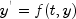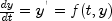JMSLTM Numerical Library 7.2.0
com.imsl.math

• All Known Subinterfaces:
Enclosing class:

`public static interface OdeAdamsGear.Function`
Public interface for user supplied function to `OdeAdamsGear` object.
• ### Method Summary

Methods
Modifier and Type Method and Description
`double[]` ```f(double t, double[] y)```
Computes the value of the functionat the given point.
• ### Method Detail

• #### f

```double[] f(double t,
double[] y)```
Computes the value of the functionat the given point.
Parameters:
`t` - a `double`, the point at which the function is to be evaluated. (Input)
`y` - a `double` array which contains the dependent variable values. (Input)
Returns:
a `double` array of length `y.length` which contains the value of the function. (Output)
JMSLTM Numerical Library 7.2.0